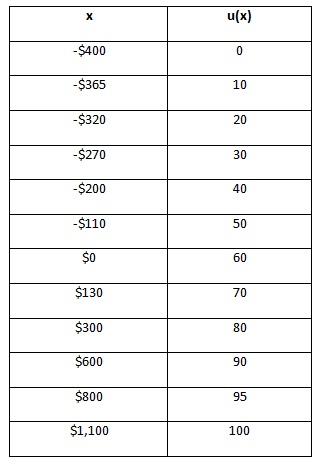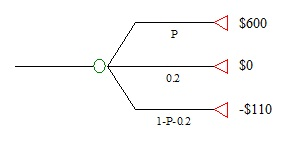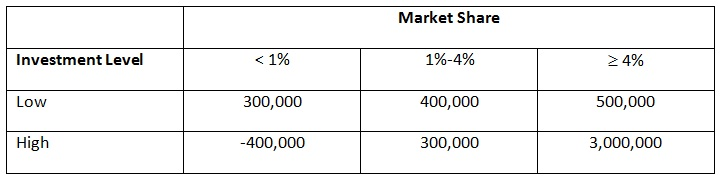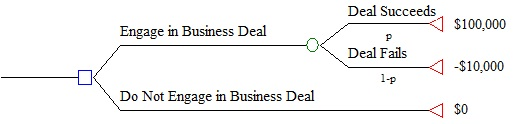Utility functions incorporate-decision makers attitude

Question: As you know, utility functions incorporate a decision maker’s attitude towards risk. Let’s assume that the following utilities were assessed for Stephanie Parker.Use these utilities to answer the following questions.

a) What is the monetary certainty equivalent for the following gamble: gain \$130 with probability 0.4, lose \$320 with probability 0.6.

b) What is the risk premium in a)? Explain the concept of a risk premium in addition to calculating its value for a).

c) What probability P would make Stephanie become indifferent between getting \$130 for sure or taking the following gamble?Question:

a) The marketing department of a vitamin water company wishes to determine the maximum expected payoff from introducing a new strawberry drink. What decision, in terms of choosing the best investment level, should the marketing department make using the payoff table below? Assume that the probability that the market share is less than 1% is 0.2, that the probability that market share is between 1 and 4% is 0.5, and that the probability that market share is at least 4% is 0.3. Assume that the marketing department is risk neutral.b) What is the maximum amount of money the company should spend to get more information about the market share?

Question: Here is a basic risky decision problem:Using the template below, sketch the results of a sensitivity analysis on P(Deal Succeeds) for a risk-neutral decision maker. How high does P(Deal Succeeds) have to before the decision maker should engage in the business deal?

#### Solution Preview :

##### Reference No:- TGS0104

Now Priced at \$45 (50% Discount)

Recommended (92%)

Rated (4.4/5)